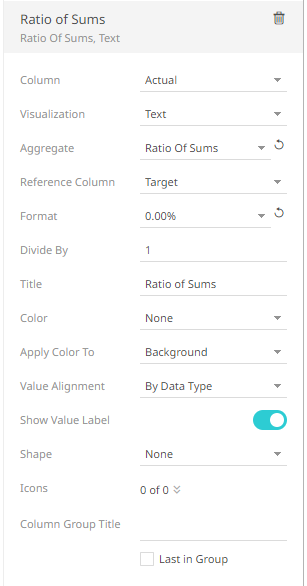Altair® Panopticon

### Ratio of Sums

The comparison between the sum of a selected measure divided by the sum of the selected reference measure.

The formula:

Ratio of Sums = sum(selected measure) / sum(selected reference measure)

Sample

Given the sample data:

 Region Store Actual Target North A \$1,300 \$2,000 North B \$750 \$1,000 North C \$2,100 \$3,000 South D \$4,700 \$4,000 South E \$2,000 \$2,000

Sample fields

Creating a Table visualization with Breakdowns Region and Store with subtotals and grand totals produces:

 Region Store Actual Target North A \$1,300 \$2,000 B \$750 \$1,000 C \$2,100 \$3,000 North Total \$4,150 \$6,000 South D \$4,700 \$4,000 E \$2,000 \$2,000 South Total \$6,700 \$6,000 Grand Total \$10,850 \$12,000

Setting the Column to Actual and the Reference Column to Target with the format set to 0.00% results to the following Ratio of Sums values:Region Store Actual Target Ratio of Sums North A \$1,300 \$2,000 65.00% B \$750 \$1,000 75.00% C \$2,100 \$3,000 70.00% North Total \$4,150 \$6,000 69.17% South D \$4,700 \$4,000 117.50% E \$2,000 \$2,000 100.00% South Total \$6,700 \$6,000 111.67% Grand Total \$10,850 \$12,000 90.42%

The results per row

Computation details:

 North A: \$1,300 / \$2,000 = 65.00% South D: \$4,700 / \$4,000 = 117.50% North B: \$750 / \$1,000 = 75.00% South E: \$2,000 / \$2,000 = 100.00% North C: \$2,100 / \$3,000 = 70.00% South Total: \$6,700 / \$6,000 = 111.67% North Total: \$4,150 / \$6,000 = 69.17% Grand Total: \$10,850 / \$12,000 = 90.42%

Collapsing the North region results to the following Ratio of Sums  values:

 Region Store Actual Target Ratio of Sums North Total \$4,150 \$6,000 69.17% South D \$4,700 \$4,000 117.50% E \$2,000 \$2,000 100.00% South Total \$6,700 \$6,000 111.67% Grand Total \$10,850 \$12,000 90.42%

The results per row

The rest of the computation details are the same except for the collapsed North region:

North = (\$1,300 + \$750 + \$2,100) / (\$2,000 + \$1,000 + \$3,000) = 69.17%

Or

North = \$4,150 / \$6,000 = 69.17%

Collapsing the South region results to the following Ratio values:

 Region Actual Target Ratio of Sums North \$4,150 \$6,000 69.17% South \$6,700 \$6,000 111.67% Grand Total \$10,850 \$12,000 90.42%

The results per row

The computation details for the collapsed South region:

South = (\$4,700 + \$2,000) / (\$4,000 + \$2,000) = 111.67%

Or

South = \$6,700 / \$6,000 = 111.67%